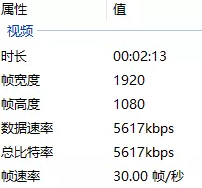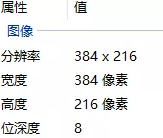﻿ 利用Python轻松实现视频转GIF动图_python_脚本之家
python# 利用Python轻松实现视频转GIF动图

## 前言## 1. 准备工作

https://zulko.github.io/moviepy/

```pip install moviepy
```

## 2. 初探

```from moviepy.editor import *

video_path = "F:\PythonCool\视频\【咒术回战】第20集五条悟帅的有些过分了.mp4"
video = VideoFileClip(video_path)
clip = (video.subclip((2,4.5),(2,6.0))
.resize(0.2))
clip.write_gif("动图搞定了.gif",fps=8)
````subclip((2,4.5),(2,6.0)`选取的是整个视频从2.45秒到2.60秒

`resize(0.2)`尺寸大小改为原始大小的0.2也就是1/5大小（毕竟动图尺寸大小其文件大小也太大）

`write_gif("动图搞定了.gif",fps=8)`保存gif的时候将帧率调整为了8## 3. 截取区域转动图

```video.crop(
x1=None,
y1=None,
x2=None,
y2=None,
width=None,
height=None,
x_center=None,
y_center=None,
)
```

`x1``y1`：矩形区域左上角坐标

`x2``y2`：矩形区域右下角坐标

`width``height`：是宽度和高度

`x_center``y_center`：表示x1的坐标为x_center-width/2，x2的坐标为x_center+width/2，y_center类似处理

```crop = (video.subclip((2,4.5),(2,6.0))
.resize(0.2)
.crop(x1=70,y1=0, x2=310,y2=216))
crop.write_gif("裁剪.gif",fps=8)
````video.resize(0.2).preview()`

## 4. 固定区域转动图

```snapshot = (crop
# 截图区域
.crop(x1=100,y1=190, x2=140,y2=216)
# 截取的时间段（1秒的时候）
.to_ImageClip(1)
# 设置位置（左上角，和截取区域的左上角一致）
.set_position((100, 190))
.set_duration(crop.duration))
# 合并
composition = CompositeVideoClip([crop, snapshot])
composition.write_gif('覆盖.gif', fps=8)
```## 5. 添加自定义文本

```from moviepy.video.VideoClip import TextClip

# 蒙版
ColorClip((40, 20), (0, 0, 0))
# 设置位置（同第4节）
.set_pos((100, 190))
.set_duration(crop.duration)
)
# 文字
text = (TextClip("搞定啦",
fontsize=30, color='white',
font='SimHei', interline=-25)
.set_pos((80, 160))
.set_duration(crop.duration))